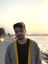Search
•Inan Evin

# #11 Lina Engine: Math Library - Part 2 Quaternions

So previously I have talked briefly about the Math and Vector3 class implementations. Honestly, there were not much to see. In this post, I would be talking about the quaternions and matrices, that will build the base of drawing calculations along with the vectors for Lina Engine.

Vectors are used in a more practical and data wise manner. They are responsible for holding coordinates, and performing operations to change these coordinates or reflect them in a different plane when necessary. However, quaternions are a little bit bigger of a deal. Quaternions are number systems that are able to extend into complex numbers. They have imaginary and a real part, and they can be represented in a programming-wise view with 4 components, x-y-z and w. We will use quaternions to perform rotations for our objects, because quaternions are mostly used to represent "transformation" in 3D space. You might ask, why would we use quaternions with 4 components to represent a transformation in a 3D space? Well, the topic goes really hard on the math, but in basic manners I will try to explain.

The idea is to represent a 3D rotation using 4 parameters. Normally, we can still use 3D vector multiplications and operations to change a state of a vector with 3 parameters, give it an angle and create a rotation out of it. Actually, this is a widely-known process called Euler Angles, and we will use it for different purposes later. However, in order to truly represent an accurate representation of a rotation on a object with 3 dimensional existence, we need to map it into a 4D hyperspace. Otherwise, there is no way to make the rotation linear. Think the quaternion's complex part as a vector of direction in 2D space. It defines the plane that we would like to base our rotation upon, and the other part as the 3D rotation to be created on that particular plane. Multiplying vectors with quaternions will result in a "space & state change" in our vectors that can be represented as rotation inside our game world. I will not be going too deep about the fundamentals of those calculations as well as the basis of Quaternions, as you might have guesses it is a huge and important concept in Mathematics and I am not a mathematician myself. So, for now, I hope I was able to deliver the importance of quaternions in a game engine, that we will mostly be dealing with 3D rendering.

Now to the implementation of a Quaternion library, I did not emplaced too much of a stuff just yet. I have basic normalization, conjugation and multiplication of Quaternion with Quaternion, and Quaternion with Vector3 just yet. The formulas are well known formulas that you can find anywhere online, and they are a pain to look at. Check the implementation below:

For now, the only important part is the multiplication method. As it might seem complex, the only thing you have to do in order to implement that is to first understand why we need quaternions, and second just the well-known formula of it. We are using these multiplication and conjugation methods in our Vector3 class to create a 3D rotation on a desired plane.

So yeah, I think that's all for this blog post, I simply wanted to explain the existence of a Quaternion class in our engine. In the next post, we will be looking at the Matrix class, at it is the backbone of creating a transformation space to draw objects in 3D space. We will be using matrices for everything, from OpenGL functions to shaders, almost everything will be working on our Matrix calculations.